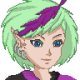-=+=- -=+=- -=+=- -=+=- -=+=- -=+=- -=+=- -=+=- -=+=- -=+=- -=+=- -=+=- -=+=- -=+=- -=+=- -=+=- -=+=- -=+=- -=+=- -=+=- -=+=- -=+=- -=+=- -=+=- -=+=- -=+=- -=+=- -=+=- -=+=- -=+=- (c) WidthPadding Industries 1987 0|591|0 -=+=- -=+=- -=+=- -=+=- -=+=- -=+=- -=+=- -=+=- -=+=- -=+=- -=+=- -=+=- -=+=- -=+=- -=+=- -=+=- -=+=- -=+=- -=+=- -=+=- -=+=- -=+=- -=+=- -=+=- -=+=- -=+=- -=+=- -=+=- -=+=- -=+=-
Socoder -> Books -> Phantasar Chronicles

Posted : Wednesday, 22 January 2020, 15:52
Tricky### Phantasar Chronicles

Aside from a coder, I am also a fantasy novel writer.
I do write in my native language, which happens to be Dutch, but I did a quick translation of a chapter I wrote, and I'll share it with you...
please note, errors can still be there, and if I can ever get a professional translator it may be possible they come up with a different translation.
But perhaps this can give you a little indication already of my work... At least, I hope so...Check it out here